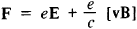# Lorentz Force

(redirected from Lorenz force)
Also found in: Dictionary, Thesaurus.

## Lorentz force

[′lȯr‚ens ‚fȯrs]
(electromagnetism)
The force on a charged particle moving in electric and magnetic fields, equal to the particle's charge times the sum of the electric field and the cross product of the particle's velocity with the magnetic flux density.

## Lorentz Force

the force acting on a charged particle moving in an electromagnetic field. The formula for the Lorentz force F was first derived by H. A. Lorentz as a result of a generalization of experiments and has the formwhere e is the charge of the particle, E is the electric field intensity, B is the magnetic induction, v is the velocity of the charged particle with respect to the coordinate system in which the quantities F, E, and B are calculated, and c is the velocity of light in a vacuum. The formula is valid for any values of the velocity of the charged particle. It is a very important relationship of electrodynamics since it makes it possible to link the electromagnetic field equations with the equations of motion of charged particles.

The first term in the right-hand side of the formula is the force acting on a charged particle in an electric field and the second term is the force in a magnetic field. The magnetic part of the Lorentz force is proportional to the vector product of v and B, that is, it is perpendicular to the velocity of the particle (the direction of its motion) and the magnetic induction vector. Consequently, it does not perform mechanical work and only curves the trajectory of the particle’s motion without changing its energy. The value of this part of the Lorentz force is equal to (ev/c)B sin a, where a is the angle between the vectors v and B; the factor 1/c is related to the choice of units of measurement —it is assumed that all quantities are measured in the absolute (Gaussian) system of units (in the International System of Units this factor is absent). Thus, the magnetic part of the Lorentz force is at a maximum if the direction of the particle’s motion forms a right angle with the direction of the magnetic field and is equal to 0 if the particle moves in the direction of the field.

In a vacuum in a constant uniform magnetic field (B = H, where H is the field intensity), a charged particle moves under the influence of the Lorentz force (its magnetic part) in a spiral path with a velocity v that is constant in magnitude. Here, its motion consists of a uniform rectilinear motion along the direction of the magnetic field H (with a velocity v that is equal to the component of the particle’s velocity v in the direction of H) and a uniform rotational motion in a plane perpendicular to H (with a velocity v1 that is equal to the component of v in the direction perpendicular to H). The projection of the trajectory of the particle’s motion on a plane perpendicular to H is a circle of radius R = cmv1/eH, and the frequency of rotation is equal to ω = eH/mc (the cyclotron frequency). The axis of the spiral path coincides with the direction of H, and the center of the circle is shifted along the field’s line of force.

If the electric field E is not equal to zero, then the motion is more complex. A shift, known as drift, of the center of the particle’s rotation perpendicular to the field H occurs. The direction of the drift is given by the vector [E H] and is independent of the sign of the charge. For the simplest case of crossed fields (E ┴ H), the rate of drift u is equal to u = cE/H.

The action of a magnetic field on moving charged particles leads to redistribution of current over the cross section of a conductor. This is manifested in various thermomagnetic and galvanomagnetic phenomena, such as the Nernst-Ettinghausen effect and the Hall effect.

### REFERENCES

Lorentz, H. A. Teoriia elektronov i ee primenenie k iavleniiam sveta i teplovogo izlucheniia, 2nd ed. Moscow, 1953. (Translated from English.)
Tamm, I. E. Osnovy teorii elektrichestva, 7th ed. Moscow, 1957.
Feynman, R., R. Leighton, and M. Sands, fasc. 6. Feinmanovskie lektsii po fizike. Moscow, 1966. (Translated from English.)
Site: Follow: Share:
Open / Close